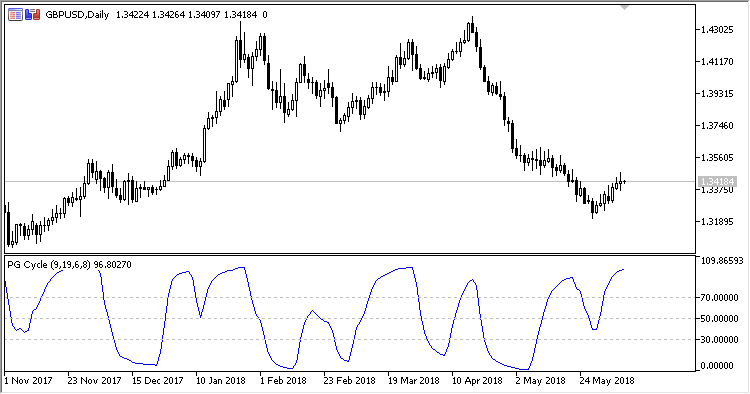# PGC – indicator for MetaTrader 5

Oscillator indicating the cyclic changes in the market.

• First MA period – first EMA period;
• Second MA period – second EMA period;
• Smoothing period – smoothing period;
• RSI period – RSI calculation period;
• Applied price – the calculation price;
• Overbought level – overbought level;
• Oversold level.

Calculation:

PGC =100.0 – 100.0 / (1.0+RS)

where:

```if AvgDec != 0
RS = AvgInc / AvgDec
otherwise
RS = 0
AvgDec = EMA(Dec, RSI Period)
AvgInc = EMA(Inc, RSI Period)
If Z3 > PrevZ3
Inc = Z3 - PrevZ3, Dec = 0
If Z3 < PrevZ3
Dec = PrevZ3 - Z3, Inc = 0
```
Z3 = 2.0 * AvgMA3 – Avg2MA3
```Avg2MA3 = EMA(AvgMA3, Smoothing period)
AvgMA3 = EMA((Z1 - Z2), Smoothing period)
Z2 = 2.0 * MA2 - AvgMA2
Z1 = 2.0 * MA1 - AvgMA1
AvgMA2 = EMA(MA2, First MA period)
AvgMA1 = EMA(MA1, First MA period)
MA2 = EMA(Applied price, Second MA period)
MA1 = EMA(Applied price, First MA period)
```